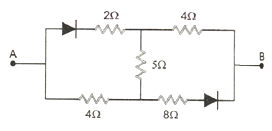Semiconductor Electronics: Materials, Devices And Simple Circuits

15 Questions | Total Attempts: 979SettingsTime: 30 Minute

• 1.
What is the possible number of crystal system?
• A.

5

• B.

7

• C.

14

• D.

16

• 2.
With fall of temperature, the forbidden energy gap of a semiconductor:
• A.

Increases

• B.

Decreases

• C.

Remains unchanged

• D.

Sometimes increases and sometimes decreases

• 3.
What would be the fundamental frequency in the ripple, if a half wave rectifier circuit is being operated from a 50 Hz mains frequency?
• A.

25 Hz

• B.

50 Hz

• C.

70.7 Hz

• D.

100 Hz

• 4.
At absolute zero, Si acts as a:
• A.

Non - metal

• B.

Metal

• C.

Insulator

• D.

None of these

• 5.
What is the equivalent resistance of the circuit across AB?
• A.

4 Ω

• B.

13 Ω

• C.

4 Ω or 13 Ω

• D.

4 Ω or 0 Ω

• 6.
The graph shown represents the I – V characteristics of a Zener diode. Which part according to you operates as a voltage regulator?
• A.

Ab

• B.

Bc

• C.

Cd

• D.

De

• 7.
For a transistor if the parameters β = 99, then the value of parameter α is:
• A.

0.9

• B.

0.99

• C.

1

• D.

9

• 8.
In the ratio of concentration of electrons that of holes in a semiconductor is 7/5 and the ratio of currents is 7/4 then what is the ratio of their drift velocity?
• A.

4/7

• B.

5/8

• C.

4/5

• D.

5/4

• 9.
What will be the output voltage of the amplifier, if in an npn transistor at common emitter configuration the current gain is given as β = 100.
• A.

10-3 V

• B.

10-1 V

• C.

100 V

• D.

10 V

• 10.
In the given figure, the current through the Zener diode is:
• A.

10 mA

• B.

6.67 mA

• C.

5 mA

• D.

3.33 mA

• 11.
The relation between α and β parameters of a transistor is given by:
• A.
• B.
• C.
• D.
• 12.
In the figure of the circuit the output y becomes zero for the inputs:
• A.

A = 1, B = 0, C = 0

• B.

A = 0, B = 1, C = 1

• C.

A = 0, B = 0, C = 0

• D.

A = 1, B = 1, C = 0

• 13.
The figure shows a logic gate circuit with two inputs A and B and the output Y. The voltage waveforms of A, B and Y are given: The logic gate is:
• A.

NAND Gate

• B.

OR Gate

• C.

AND Gate

• D.

NOR Gate

• 14.
The circuit diagram shows a logic combination with the states of outputs X, Y and Z given for inputs P, Q, R and S all at state 1. When input P and R change to state 0 with inputs Q and S still at 1, the states of outputs X, Y and Z change to:
• A.

1, 0, 0

• B.

0, 1, 0

• C.

0, 0, 1

• D.

0, 1, 1

• 15.
Two ideal diodes are connected to a battery as shown in the figure. The current supplied by the battery is:
• A.

0.75 A

• B.

Zero

• C.

0.25 A

• D.

0.5 A

Related TopicsBack to top